# Totient function

(diff) ← Older revision | Latest revision (diff) | Newer revision → (diff)

Euler totient function, Euler totient

Another frequently used named for the Euler function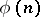, which counts the natural numbers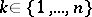that are relatively prime to.

The Carmichael conjecture on the Euler totient function states that if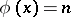, then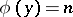for some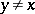; i.e. no value of the Euler function is assumed once. This has now been verified for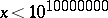, [a1].

A natural generalization of the Euler totient function is the Jordan totient function, which counts the number of-tuples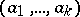,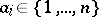, such that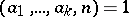. Clearly,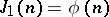.

One has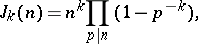whereruns over the prime numbers dividing, and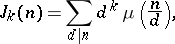whereis the Möbius function andruns over all divisors of. Forthese formulas reduce to the well-known formulas for the Euler function.

The Lehmer problem on the Euler totient function asks for the solutions of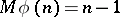,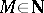, [a2]. For some results on this still (1996) largely open problem, see [a3] and the references therein. The corresponding problem for the Jordan totient function (and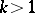) is easy, [a4]: For,if and only ifis a prime number. Moreover, ifis a prime number, then.

For much more information on the Euler totient function, the Jordan totient function and various other generalizations, see [a5], [a6].

How to Cite This Entry:
Totient function. Encyclopedia of Mathematics. URL: http://encyclopediaofmath.org/index.php?title=Totient_function&oldid=12673
This article was adapted from an original article by M. Hazewinkel (originator), which appeared in Encyclopedia of Mathematics - ISBN 1402006098. See original article